# Procedure - To show that the Area of Rhombus is half the Product of its Diagonals, Math, Class 9 Class 9 Notes | EduRev

## Class 9 : Procedure - To show that the Area of Rhombus is half the Product of its Diagonals, Math, Class 9 Class 9 Notes | EduRev

The document Procedure - To show that the Area of Rhombus is half the Product of its Diagonals, Math, Class 9 Class 9 Notes | EduRev is a part of the Class 9 Course Mathematics (Maths) Class 9.
All you need of Class 9 at this link: Class 9

Objective

To show that the area of rhombus is half the product of its diagonals.

Theory

1. A rhombus is a simple (non-self-intersecting) quadrilateral whose all four sides are of same length.

2. If a parallelogram has two consecutive sides congruent, it is a rhombus.

3. If two triangles are congruent then their areas are equal.

4. Area of a triangle = 1/2 X base X height

5. Area of a rectangle = Length X Breadth

Proof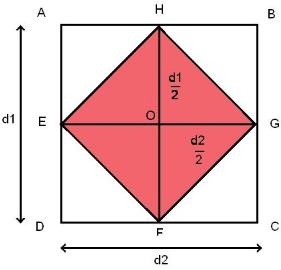In above figure EHGF is rhombus with diagonal HF (length d1) and diagonal EG (length d2)

Area of rhombus EHGF = Area of triangle EFH + Area of triangle FH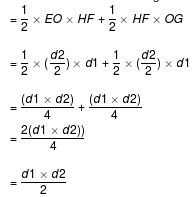= half of the product of the diagonals

Example

Find the area of the following rhombus.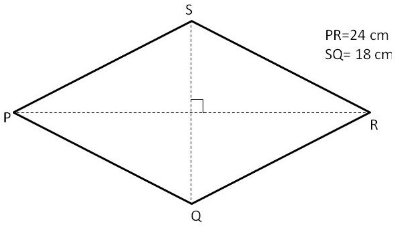Solution:

In the given figure,

PR = d1= 24 cm.

SQ = d= 18 cm.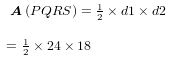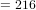So, the area of the rhombus PQRS is 216 cm2.

Offer running on EduRev: Apply code STAYHOME200 to get INR 200 off on our premium plan EduRev Infinity!

## Mathematics (Maths) Class 9

190 videos|233 docs|82 tests

,

,

,

,

,

,

,

,

,

,

,

,

,

,

,

,

,

,

,

,

,

,

,

,

,

,

,

;